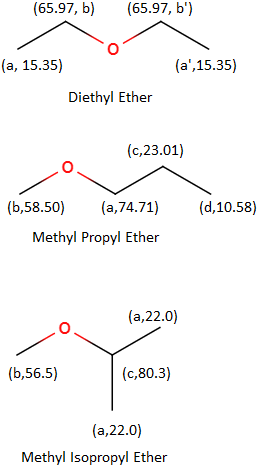# Predict the structure: an isomer of C_4H_10O that isn't an alcohol. Determine the degrees of...

## Question:

Predict the structure: an isomer of {eq}C_4H_{10}O {/eq} that isn't an alcohol. Determine the degrees of unsaturation. Assign all the peaks in the spectrum to the carbons in your predicted structure.

## Degrees of Unsaturation:

Because carbon atoms almost always have 4 bonds (to obey the octet rule), it is fairly easy to predict the chemical formulae of fully saturated carbon compounds. Take the following alkanes as an example: methane ({eq}CH_4} {/eq}), ethane ({eq}C_{2}H_{6} {/eq}), propane ({eq}C_{3}H_{8} {/eq}, butane ({eq}C_{4}H_{10} {/eq}. All of these compounds contain a number of hydrogens equal to 2n+2, where n = the number of carbon atoms. Though structures (that don't contain nitrogen) can't have more hydrogen atoms than predicted here, they can have less. Ethene, for example, has 2 fewer hydrogen atoms because of the double bond present in the compound. We would call ethene unsaturated because it does not have as many hydrogen atoms as the 2n+2 expression would predict.

Because there are 4 carbon atoms in the given formula, there should be 10 hydrogen atoms. As there are 10, the degree of unsaturation is: 10 - 10 = 0.

As there are no degrees of unsaturation, there cannot be a carbonyl. That leaves the only possible structures as ethers (because we are given the constraint that the oxygen atom cannot be an alcohol). Only three possible structures can exist and they are shown below (with names, unique carbons labeled, and C-NMR shifts):